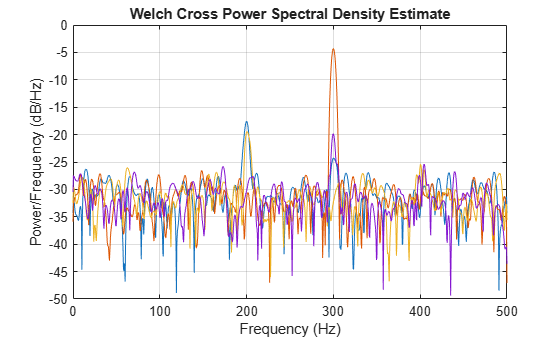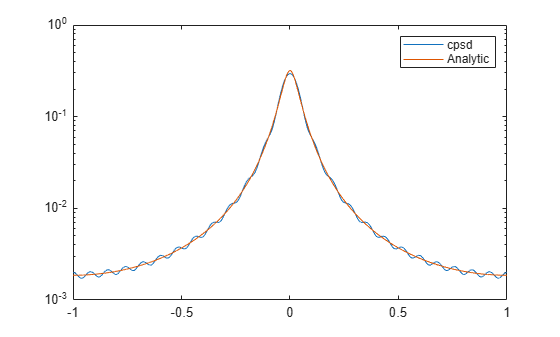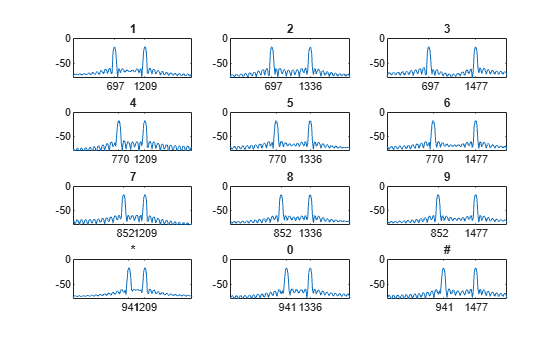# cpsd

Cross power spectral density

## Syntax

``pxy = cpsd(x,y)``
``pxy = cpsd(x,y,window)``
``pxy = cpsd(x,y,window,noverlap)``
``pxy = cpsd(x,y,window,noverlap,nfft)``
``pxy = cpsd(___,'mimo')``
``[pxy,w] = cpsd(___)``
``[pxy,f] = cpsd(___,fs)``
``[pxy,w] = cpsd(x,y,window,noverlap,w)``
``[pxy,f] = cpsd(x,y,window,noverlap,f,fs)``
``[___] = cpsd(x,y,___,freqrange)``
``cpsd(___)``

## Description

example

````pxy = cpsd(x,y)` estimates the cross power spectral density (CPSD) of two discrete-time signals, `x` and `y`, using Welch’s averaged, modified periodogram method of spectral estimation. If `x` and `y` are both vectors, they must have the same length.If one of the signals is a matrix and the other is a vector, then the length of the vector must equal the number of rows in the matrix. The function expands the vector and returns a matrix of column-by-column cross power spectral density estimates.If `x` and `y` are matrices with the same number of rows but different numbers of columns, then `cpsd` returns a three-dimensional array, `pxy`, containing cross power spectral density estimates for all combinations of input columns. Each column of `pxy` corresponds to a column of `x`, and each page corresponds to a column of `y`: `pxy(:,m,n) = cpsd(x(:,m),y(:,n))`.If `x` and `y` are matrices of equal size, then `cpsd` operates column-wise: ```pxy(:,n) = cpsd(x(:,n),y(:,n))```. To obtain a multi-input/multi-output array, append `'mimo'` to the argument list. For real `x` and `y`, `cpsd` returns a one-sided CPSD. For complex `x` or `y`, `cpsd` returns a two-sided CPSD.```
````pxy = cpsd(x,y,window)` uses `window` to divide `x` and `y` into segments and perform windowing.```
````pxy = cpsd(x,y,window,noverlap)` uses `noverlap` samples of overlap between adjoining segments.```
````pxy = cpsd(x,y,window,noverlap,nfft)` uses `nfft` sampling points to calculate the discrete Fourier transform.```

example

````pxy = cpsd(___,'mimo')` computes a multi-input/multi-output array of cross power spectral density estimates. This syntax can include any combination of input arguments from previous syntaxes.```
````[pxy,w] = cpsd(___)` returns a vector of normalized frequencies, `w`, at which the cross power spectral density is estimated.```
````[pxy,f] = cpsd(___,fs)` returns a vector of frequencies, `f`, expressed in terms of the sample rate, `fs`, at which the cross power spectral density is estimated. `fs` must be the sixth numeric input to `cpsd`. To input a sample rate and still use the default values of the preceding optional arguments, specify these arguments as empty, `[]`.```

example

````[pxy,w] = cpsd(x,y,window,noverlap,w)` returns the cross power spectral density estimates at the normalized frequencies specified in `w`.```

example

````[pxy,f] = cpsd(x,y,window,noverlap,f,fs)` returns the cross power spectral density estimates at the frequencies specified in `f`.```
````[___] = cpsd(x,y,___,freqrange)` returns the cross power spectral density estimate over the frequency range specified by `freqrange`. Valid options for `freqrange` are `'onesided'`, `'twosided'`, and `'centered'`.```

example

````cpsd(___)` with no output arguments plots the cross power spectral density estimate in the current figure window.```

## Examples

collapse all

Generate two colored noise signals and plot their cross power spectral density. Specify a length-1024 FFT and a 500-point triangular window with 50% overlap between segments.

```rng default r = randn(2e4,1); hx = fir1(30,0.2,rectwin(31)); x = filter(hx,1,r); hy = ones(1,10); y = filter(hy,1,r); win = triang(500); nov = 250; cpsd(x,y,win,nov,1024)```Generate two two-channel sinusoids sampled at 1 kHz for 1 second. The channels of the first signal have frequencies of 200 Hz and 300 Hz. The channels of the second signal have frequencies of 300 Hz and 400 Hz. Both signals are embedded in unit-variance white Gaussian noise.

```fs = 1e3; t = (0:1/fs:1-1/fs)'; q = 2*sin(2*pi*[200 300].*t); q = q+randn(size(q)); r = 2*sin(2*pi*[300 400].*t); r = r+randn(size(r));```

Compute the cross power spectral density of the two signals. Use a 256-sample Bartlett window to divide the signals into segments and window the segments. Specify 128 samples of overlap between adjoining segments and 2048 DFT points. Use the built-in functionality of `cpsd` to plot the result.

`cpsd(q,r,bartlett(256),128,2048,fs)`By default, `cpsd` works column-by-column on matrix inputs of the same size. Each channel peaks at the frequencies of the original sinusoids.

Repeat the calculation, but now append `'mimo'` to the list of arguments.

`cpsd(q,r,bartlett(256),128,2048,fs,'mimo')`When called with the `'mimo'` option, `cpsd` returns a three-dimensional array containing cross power spectral density estimates for all combinations of input columns. The estimate of the second channel of `q` and the first channel of `r` shows an enhanced peak at the common frequency of 300 Hz.

Generate two 100 Hz sinusoidal signals sampled at 1 kHz for 296 ms. One of the sinusoids lags the other by 2.5 ms, equivalent to a phase lag of π/2. Both signals are embedded in white Gaussian noise of variance 1/4².

```Fs = 1000; t = 0:1/Fs:0.296; x = cos(2*pi*t*100)+0.25*randn(size(t)); tau = 1/400; y = cos(2*pi*100*(t-tau))+0.25*randn(size(t));```

Compute and plot the magnitude of the cross power spectral density. Use the default settings for `cpsd`. The magnitude peaks at the frequency where there is significant coherence between the signals.

`cpsd(x,y,[],[],[],Fs)`Plot magnitude-squared coherence function and the phase of the cross spectrum. The ordinate at the high-coherence frequency corresponds to the phase lag between the sinusoids.

```[Cxy,F] = mscohere(x,y,[],[],[],Fs); [Pxy,F] = cpsd(x,y,[],[],[],Fs); subplot(2,1,1) plot(F,Cxy) title('Magnitude-Squared Coherence') subplot(2,1,2) plot(F,angle(Pxy)) hold on plot(F,2*pi*100*tau*ones(size(F)),'--') hold off xlabel('Hz') ylabel('\Theta(f)') title('Cross Spectrum Phase')```Generate two $N$-sample exponential sequences, ${x}_{a}={a}^{n}$ and ${x}_{b}={b}^{n}$, with $n\ge 0$. Specify $a=0.8$, $b=0.9$, and a small $N$ to see finite-size effects.

```N = 10; n = 0:N-1; a = 0.8; b = 0.9; xa = a.^n; xb = b.^n;```

Compute and plot the cross power spectral density of the sequences over the complete interval of normalized frequencies, $\left[-\pi ,\pi \right]$. Specify a rectangular window of length $N$ and no overlap between segments.

```w = -pi:1/1000:pi; wind = rectwin(N); nove = 0; [pxx,f] = cpsd(xa,xb,wind,nove,w);```

The cross power spectrum of the two sequences has an analytic expression for large $N$:

`$R\left(\omega \right)=\frac{1}{1-a{e}^{-j\omega }}\phantom{\rule{0.16666666666666666em}{0ex}}\frac{1}{1-b{e}^{j\omega }}.$`

Convert this expression to a cross power spectral density by dividing it by $2\pi N$. Compare the results. The ripple in the `cpsd` result is a consequence of windowing.

```nfac = 2*pi*N; X = 1./(1-a*exp(-1j*w)); Y = 1./(1-b*exp( 1j*w)); R = X.*Y/nfac; semilogy(f/pi,abs(pxx)) hold on semilogy(w/pi,abs(R)) hold off legend('cpsd','Analytic')```Repeat the calculation with $N=25$. The curves agree to six figures for $N$ as small as 100.

```N = 25; n = 0:N-1; xa = a.^n; xb = b.^n; wind = rectwin(N); [pxx,f] = cpsd(xa,xb,wind,nove,w); R = X.*Y/(2*pi*N); semilogy(f/pi,abs(pxx)) hold on semilogy(w/pi,abs(R)) hold off legend('cpsd','Analytic')```Use cross power spectral density to identify a highly corrupted tone.

The sound signals generated when you dial a number or symbol on a digital phone are sums of sinusoids with frequencies taken from two different groups. Each pair of tones contains one frequency of the low group (697 Hz, 770 Hz, 852 Hz, or 941 Hz) and one frequency of the high group (1209 Hz, 1336 Hz, or 1477 Hz).Generate signals corresponding to all the symbols. Sample each tone at 4 kHz for half a second. Prepare a reference table.

```fs = 4e3; t = 0:1/fs:0.5-1/fs; nms = ['1';'2';'3';'4';'5';'6';'7';'8';'9';'*';'0';'#']; ver = [697 770 852 941]; hor = [1209 1336 1477]; v = length(ver); h = length(hor); for k = 1:v for l = 1:h idx = h*(k-1)+l; tone = sum(sin(2*pi*[ver(k);hor(l)].*t))'; tones(:,idx) = tone; end end```

Plot the Welch periodogram of each signal and annotate the component frequencies. Use a 200-sample Hamming window to divide the signals into non-overlapping segments and window the segments.

```[pxx,f] = pwelch(tones,hamming(200),0,[],fs); for k = 1:v for l = 1:h idx = h*(k-1)+l; ax = subplot(v,h,idx); plot(f,10*log10(pxx(:,idx))) ylim([-80 0]) title(nms(idx)) tx = [ver(k);hor(l)]; ax.XTick = tx; ax.XTickLabel = int2str(tx); end end```A signal produced by dialing the number 8 is sent through a noisy channel. The received signal is so corrupted that the number cannot be identified by inspection.

```mys = sum(sin(2*pi*[ver(3);hor(2)].*t))'+5*randn(size(t')); % To hear, type soundsc(mys,fs)```

Compute the cross power spectral density of the corrupted signal and the reference signals. Window the signals using a 512-sample Kaiser window with shape factor β = 5. Plot the magnitude of each spectrum.

```[pxy,f] = cpsd(mys,tones,kaiser(512,5),100,[],fs); for k = 1:v for l = 1:h idx = h*(k-1)+l; ax = subplot(v,h,idx); plot(f,10*log10(abs(pxy(:,idx)))) ylim([-80 0]) title(nms(idx)) tx = [ver(k);hor(l)]; ax.XTick = tx; ax.XTickLabel = int2str(tx); end end```The digit in the corrupted signal has the spectrum with the highest peaks and the highest RMS value.

```[~,loc] = max(rms(abs(pxy))); digit = nms(loc)```
```digit = '8' ```

## Input Arguments

collapse all

Input signals, specified as vectors or matrices.

Example: `cos(pi/4*(0:159))+randn(1,160)` specifies a sinusoid embedded in white Gaussian noise.

Data Types: `single` | `double`
Complex Number Support: Yes

Window, specified as an integer or as a row or column vector. Use `window` to divide the signal into segments.

• If `window` is an integer, then `cpsd` divides `x` and `y` into segments of length `window` and windows each segment with a Hamming window of that length.

• If `window` is a vector, then `cpsd` divides `x` and `y` into segments of the same length as the vector and windows each segment using `window`.

If the length of `x` and `y` cannot be divided exactly into an integer number of segments with `noverlap` overlapping samples, then the signals are truncated accordingly.

If you specify `window` as empty, then `cpsd` uses a Hamming window such that `x` and `y` are divided into eight segments with `noverlap` overlapping samples.

For a list of available windows, see Windows.

Example: `hann(N+1)` and `(1-cos(2*pi*(0:N)'/N))/2` both specify a Hann window of length `N` + 1.

Data Types: `single` | `double`

Number of overlapped samples, specified as a positive integer.

• If `window` is scalar, then `noverlap` must be smaller than `window`.

• If `window` is a vector, then `noverlap` must be smaller than the length of `window`.

If you specify `noverlap` as empty, then `cpsd` uses a number that produces 50% overlap between segments. If the segment length is unspecified, the function sets `noverlap` to ⌊N/4.5⌋, where N is the length of the input and output signals.

Data Types: `double` | `single`

Number of DFT points, specified as a positive integer. If you specify `nfft` as empty, then `cpsd` sets the parameter to max(256,2p), where p = ⌈log2 N for input signals of length N.

Data Types: `single` | `double`

Sample rate, specified as a positive scalar. The sample rate is the number of samples per unit time. If the unit of time is seconds, then the sample rate has units of Hz.

Normalized frequencies, specified as a row or column vector with at least two elements. Normalized frequencies are in rad/sample.

Example: `w = [pi/4 pi/2]`

Data Types: `double` | `single`

Frequencies, specified as a row or column vector with at least two elements. The frequencies are in cycles per unit time. The unit time is specified by the sample rate, `fs`. If `fs` has units of samples/second, then `f` has units of Hz.

Example: `fs = 1000; f = [100 200]`

Data Types: `double` | `single`

Frequency range for cross power spectral density estimate, specified as `'onesided'`, `'twosided'`, or `'centered'`. The default is `'onesided'` for real-valued signals and `'twosided'` for complex-valued signals.

• `'onesided'` — Returns the one-sided estimate of the cross power spectral density of two real-valued input signals, `x` and `y`. If `nfft` is even, `pxy` has `nfft`/2 + 1 rows and is computed over the interval [0,π] rad/sample. If `nfft` is odd, `pxy` has (`nfft` + 1)/2 rows and the interval is [0,π) rad/sample. If you specify `fs`, the corresponding intervals are [0,`fs`/2] cycles/unit time for even `nfft` and [0,`fs`/2) cycles/unit time for odd `nfft`.

• `'twosided'` — Returns the two-sided estimate of the cross power spectral density of two real-valued or complex-valued input signals, `x` and `y`. In this case, `pxy` has `nfft` rows and is computed over the interval [0,2π) rad/sample. If you specify `fs`, the interval is [0,`fs`) cycles/unit time.

• `'centered'` — Returns the centered two-sided estimate of the cross power spectral density of two real-valued or complex-valued input signals, `x` and `y`. In this case, `pxy` has `nfft` rows and is computed over the interval (–π,π] rad/sample for even `nfft` and (–π,π) rad/sample for odd `nfft`. If you specify `fs`, the corresponding intervals are (–`fs`/2, `fs`/2] cycles/unit time for even `nfft` and (–`fs`/2, `fs`/2) cycles/unit time for odd `nfft`.

## Output Arguments

collapse all

Cross power spectral density, returned as a vector, matrix, or three-dimensional array.

Normalized frequencies, returned as a real-valued column vector.

• If `pxy` is one-sided, `w` spans the interval [0,π] when `nfft` is even and [0,π) when `nfft` is odd.

• If `pxy` is two-sided, `w` spans the interval [0,2π).

• If `pxy` is DC-centered, `w` spans the interval (–π,π] when `nfft` is even and (–π,π) when `nfft` is odd.

Data Types: `double` | `single`

Frequencies, returned as a real-valued column vector.

Data Types: `double` | `single`

collapse all

### Cross Power Spectral Density

The cross power spectral density is the distribution of power per unit frequency and is defined as

`${P}_{xy}\left(\omega \right)=\sum _{m=-\infty }^{\infty }{R}_{xy}\left(m\right){e}^{-j\omega m}.$`

The cross-correlation sequence is defined as

`${R}_{xy}\left(m\right)=E\left\{{x}_{n+m}{y}_{n}^{\ast }\right\}=E\left\{{x}_{n}{y}_{n-m}^{\ast }\right\},$`

where xn and yn are jointly stationary random processes, –∞ < n < ∞, $-\infty , and E {· } is the expected value operator.

## Algorithms

`cpsd` uses Welch’s averaged, modified periodogram method of spectral estimation.

 Oppenheim, Alan V., Ronald W. Schafer, and John R. Buck. Discrete-Time Signal Processing. 2nd Ed. Upper Saddle River, NJ: Prentice Hall, 1999.

 Rabiner, Lawrence R., and B. Gold. Theory and Application of Digital Signal Processing. Englewood Cliffs, NJ: Prentice-Hall, 1975, pp. 414–419.

 Welch, Peter D. “The Use of the Fast Fourier Transform for the Estimation of Power Spectra: A Method Based on Time Averaging Over Short, Modified Periodograms.” IEEE® Transactions on Audio and Electroacoustics, Vol. AU-15, June 1967, pp. 70–73.